# FIR Rate Conversion

Upsample, filter, and downsample input signals

• Library:
• DSP System Toolbox / Filtering / Multirate Filters

•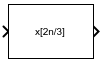## Description

The FIR Rate Conversion block resamples the discrete-time input such that its sample period is M/L times the input sample period (Tsi). M is the integer value you specify for the Decimation factor parameter, and L is the integer value you specify for the Interpolation factor parameter. The block treats each column of the input as a separate channel and resamples the data in each channel independently over time.

Conceptually, the rate converter combines an FIR interpolator followed by an FIR decimator. The following schematic contains an upsampler, a combined anti-imaging and anti-aliasing FIR filter, and a downsampler. To design an FIR filter which acts as a combined anti-imaging and anti-aliasing FIR filter, use the `designMultirateFIR` function.

The rate converter does the following:

1. Upsamples the input to a higher rate by inserting L`1` zeros between input samples.

2. Passes the upsampled data through an FIR filter.

3. Downsamples the filtered data to a lower rate by discarding M-`1` consecutive samples following each sample that the block retains.Note that the actual block algorithm implements a polyphase structure, an efficient equivalent of the combined system depicted in the diagram. For more details, see Algorithms.

## Ports

### Input

expand all

Input signal, specified as a vector or a matrix.

When the block input is fixed point, all internal data types are signed fixed point.

Data Types: `single` | `double` | `int8` | `int16` | `int32` | `int64` | `uint8` | `uint16` | `uint32` | `uint64` | `fixed point`
Complex Number Support: Yes

### Output

expand all

Rate converted signal, returned as a vector or a matrix.

When the Rate options parameter is set to:

• `Enforce single-rate processing` –– The output frame size Ko is L/M times the input frame size Ki, where L is the interpolation factor and M is the decimation factor.

Ko = (L/MKi

The output signal rate in Simulink® equals the input signal rate.

Fo = Fi

• `Allow multirate processing` –– The output frame size equals the input frame size.

Ko = Ki

The output signal rate in Simulink is L/M times the input signal rate.

Fo = (L/MFi

All blocks connected to the output operate at Fo, and all blocks connected to the input operate at Fi.

Data Types: `single` | `double` | `int8` | `int16` | `int32` | `int64` | `uint8` | `uint16` | `uint32` | `uint64` | `fixed point`
Complex Number Support: Yes

## Parameters

expand all

The FIR Rate Conversion block can operate in three different modes. Select the mode in the Coefficient source group box.

• Dialog parameters — Enter information about the filter, such as FIR filter coefficients in the block dialog box.

• Filter object — Specify the filter using a `dsp.FIRRateConverter` System object™.

• Auto (default) — The block determines the filter coefficients.

The settings in the FIR Rate Conversion block dialog box change based on the mode selected.

### Main Tab

Specify the interpolation factor, L, as a positive integer. The block upsamples the signal by this value before filtering it.

#### Dependencies

To enable this parameter, set Coefficient source to either Dialog parameters or Auto.

Data Types: `single` | `double` | `int8` | `int16` | `int32` | `int64` | `uint8` | `uint16` | `uint32` | `uint64`

Specify the FIR filter coefficients in descending powers of z. By default, the block uses the `designMultirateFIR(3,2)` function to compute the filter coefficients.

#### Dependencies

To enable this parameter, set Coefficient source to Dialog parameters.

Data Types: `single` | `double` | `int8` | `int16` | `int32` | `int64` | `uint8` | `uint16` | `uint32` | `uint64`
Complex Number Support: Yes

Specify the decimation factor, M, as a positive integer. The block downsamples the signal by this value after filtering it.

#### Dependencies

To enable this parameter, set Coefficient source to either Dialog parameters or Auto.

Data Types: `single` | `double` | `int8` | `int16` | `int32` | `int64` | `uint8` | `uint16` | `uint32` | `uint64`

Specify whether to enforce single-rate processing or allow multirate processing.

• `Enforce single-rate processing` –– The output frame size Ko is L/M times the input frame size Ki, where L is the interpolation factor and M is the decimation factor.

Ko = (L/MKi

The output signal rate in Simulink equals the input signal rate.

Fo = Fi

• `Allow multirate processing` –– The output frame size equals the input frame size.

Ko = Ki

The output signal rate in Simulink is L/M times the input signal rate.

Fo = (L/MFi

All blocks connected to the output operate at Fo, and all blocks connected to the input operate at Fi.

Specify the multirate filter object that you want the block to implement. The specified filter object must be a `dsp.FIRRateConverter` System object.

You can define the System object in the block mask or in a MATLAB® workspace variable.

For information on creating System objects, see Define Basic System Objects.

#### Dependencies

This parameter appears when Coefficient source is set to Filter object.

Select this parameter to open the Filter Visualization Tool, `fvtool`, and display the magnitude response of the FIR filter. The response is based on the parameters selected in the block dialog box. Changes made to these parameters update `fvtool`.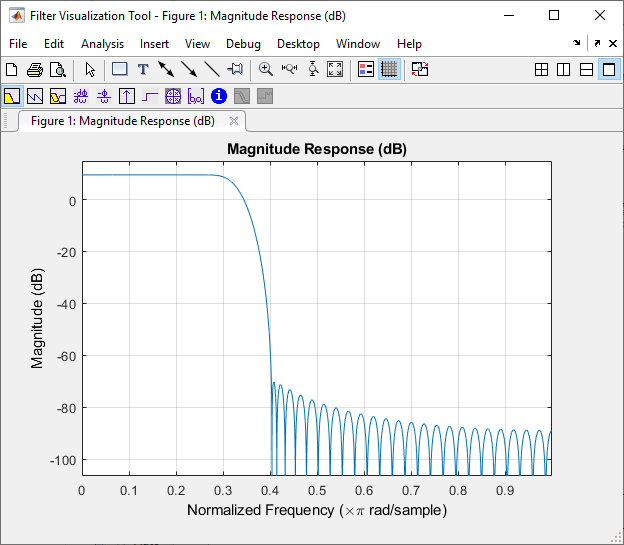To update the magnitude response while `fvtool` is running, modify the block parameters and click .

To view the magnitude response and phase response simultaneously, click the button on the toolbar.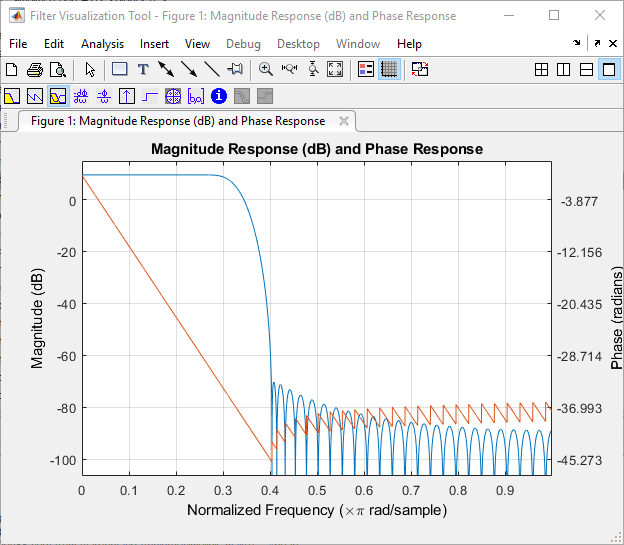### Data Types Tab

When Coefficient source is set to Filter object, the fixed-point settings of the filter object specified on the Main tab are displayed on the Data Types tab. You cannot change these settings directly on the block dialog box. To change the fixed-point settings, you must edit the filter object.

For more information on System objects, see the What Are System Objects?.

When Coefficient source is set to Auto, the block chooses the filter coefficients automatically. For more information on the filter design algorithm that the block uses, see Specify FIR Filter Coefficients.

Specify the rounding mode for fixed-point operations as:

• `Floor`

• `Ceiling`

• `Convergent`

• `Nearest`

• `Round`

• `Simplest`

• `Zero`

For more details, see Rounding Modes.

The filter coefficients do not obey this parameter and always round to `Nearest`.

Note

The Rounding mode and Saturate on integer overflow parameters have no effect on numerical results when all these conditions are met:

• Product output data type is ```Inherit: Inherit via internal rule```.

• Accumulator data type is ```Inherit: Inherit via internal rule```.

• Output data type is ```Inherit: Same as accumulator```.

With these data-type settings, the block operates in a full-precision mode.

#### Dependencies

To enable this parameter, set Coefficient source to either Dialog parameters or Auto.

Select this parameter to saturate the result of the fixed-point operation. Clear this parameter to wrap the result of the fixed-point operation. For details on saturate and wrap, see Overflow Handling for fixed-point operations.

Note

The Rounding mode and Saturate on integer overflow parameters have no effect on numeric results when all these conditions are met:

• Product output data type is ```Inherit: Inherit via internal rule```.

• Accumulator data type is ```Inherit: Inherit via internal rule```.

With these data-type settings, the block operates in a full-precision mode.

#### Dependencies

This parameter is editable only when Coefficient source is set to either Dialog parameters or Auto.

Coefficients specifies the data type of the filter coefficients.

• `Inherit: Same word length as input` –– The block inherits the word length of the coefficients from the fixed-point input. The fraction length is determined based on the coefficient values in order to obtain the best possible precision.

• `fixdt(1,16)` –– The coefficients data type is a signed, binary-point, scaled, fixed-point data type with a word length of 16 bits.

• `fixdt(1,16,0)` –– The coefficients data type is a signed, binary-point, scaled, fixed-point data type with a word length of 16 bits and a fraction length of 0.

Alternatively, you can set the Coefficients data type by using the Data Type Assistant. To use the assistant, click the button.

For more information on the data type assistant, see Specify Data Types Using Data Type Assistant (Simulink).

For a diagrammatic representation of how this block uses the filter coefficients data type, see Fixed Point.

#### Dependencies

This parameter is editable only when Coefficient source is set to either Dialog parameters or Auto.

Specify the minimum value of the filter coefficients. Simulink uses this minimum value to perform automatic scaling of fixed-point data types.

Specify the maximum value of the filter coefficients. Simulink uses this maximum value to perform automatic scaling of fixed-point data types.

Product output specifies the data type of the output of a product operation in the FIR Rate Conversion block.

• `Inherit: Inherit via internal rule` — The block inherits the product output data type based on an internal rule. For more information on this rule, see Inherit via Internal Rule.

• `Inherit: Same as input` — The block specifies the product output data type to be the same as the input data type.

• `fixdt(1,16,0)` — The block specifies a signed, binary-point, scaled, fixed-point data type with a word length of 16 bits and a fraction length of 0.

Alternatively, you can set the Product output data type by using the Data Type Assistant. To use the assistant, click the button.

For more information on the data type assistant, see Specify Data Types Using Data Type Assistant (Simulink).

For a diagrammatic representation of how this block uses the product output data type, see Fixed Point.

#### Dependencies

This parameter is editable only when Coefficient source is set to either Dialog parameters or Auto.

Accumulator specifies the data type of the output of an accumulation operation in the FIR Rate Conversion block. For illustrations on how this block uses the accumulator data type, see Fixed Point.

• `Inherit: Inherit via internal rule` — The block inherits the accumulator data type based on an internal rule. For more information on this rule, see Inherit via Internal Rule.

• `Inherit: Same as input` — The block specifies the accumulator data type to be the same as the input data type.

• `Inherit: Same as product output` — The block specifies the accumulator data type to be the same as the product output data type.

• `fixdt(1,16,0)` — The block specifies a signed, binary-point, scaled, fixed-point data type with a word length of 16 bits and a fraction length of 0.

Alternatively, you can set the Accumulator data type by using the Data Type Assistant. To use the assistant, click the button.

For more information on the data type assistant, see Specify Data Types Using Data Type Assistant (Simulink).

#### Dependencies

This parameter is editable only when Coefficient source is set to either Dialog parameters or Auto.

Output specifies the data type of the output of the FIR Rate Conversion block.

• `Inherit: Same as input` — The block specifies the output data type to be the same as the input data type.

• `Inherit: Same as product output` — The block specifies the output data type to be the same as the product output data type.

• `Inherit: Same as accumulator` — The block specifies the output data type to be the same as the accumulator data type.

• `fixdt(1,16,0)` — The block specifies a signed, binary-point, scaled, fixed-point data type with a word length of 16 bits and a fraction length of 0.

Alternatively, you can set the Output data type by using the Data Type Assistant. To use the assistant, click the button.

For more information on the data type assistant, see Specify Data Types Using Data Type Assistant (Simulink).

For a diagrammatic representation of how this block uses the output data type, see Fixed Point.

#### Dependencies

This parameter is editable only when Coefficient source is set to either Dialog parameters or Auto.

Specify the minimum value the block can output. Simulink uses this minimum value to perform:

• Automatic scaling of fixed-point data types.

Specify the maximum value the block can output. Simulink uses this maximum value to perform:

• Automatic scaling of fixed-point data types.

Select this parameter to prevent the fixed-point tools from overriding the data types you specify on the block dialog box.

#### Dependencies

This parameter appears only when Coefficient source is set to either Dialog parameters or Auto.

## Block Characteristics

 Data Types `double` | `fixed point` | `integer` | `single` Direct Feedthrough `no` Multidimensional Signals `no` Variable-Size Signals `no` Zero-Crossing Detection `no`

expand all

## Algorithms

The FIR rate converter is implemented efficiently using a polyphase structure.

To derive the polyphase structure, start with the transfer function of the FIR filter: This FIR filter is a combined anti-imaging and anti-aliasing filter.

`$H\left(z\right)={b}_{0}+{b}_{1}{z}^{-1}+...+{b}_{N}{z}^{-N}$`

N+1 is the length of the FIR filter.

You can rearrange this equation as follows:

`$H\left(z\right)=\begin{array}{c}\left({b}_{0}+{b}_{L}{z}^{-L}+{b}_{2L}{z}^{-2L}+..+{b}_{N-L+1}{z}^{-\left(N-L+1\right)}\right)+\\ {z}^{-1}\left({b}_{1}+{b}_{L+1}{z}^{-L}+{b}_{2L+1}{z}^{-2L}+..+{b}_{N-L+2}{z}^{-\left(N-L+1\right)}\right)+\\ \begin{array}{c}⋮\\ {z}^{-\left(L-1\right)}\left({b}_{L-1}+{b}_{2L-1}{z}^{-L}+{b}_{3L-1}{z}^{-2L}+..+{b}_{N}{z}^{-\left(N-L+1\right)}\right)\end{array}\end{array}$`

L is the number of polyphase components, and its value equals the interpolation factor that you specify.

You can write this equation as:

`$H\left(z\right)={E}_{0}\left({z}^{L}\right)+{z}^{-1}{E}_{1}\left({z}^{L}\right)+...+{z}^{-\left(L-1\right)}{E}_{L-1}\left({z}^{L}\right)$`

E0(zL), E1(zL), ..., EL-1(zL) are polyphase components of the FIR filter H(z).

Conceptually, the FIR rate converter contains an upsampler, followed by a combined anti-imaging, anti-aliasing FIR filter H(z), which is followed by a downsampler.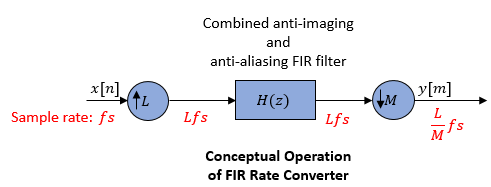Replace H(z) with its polyphase representation.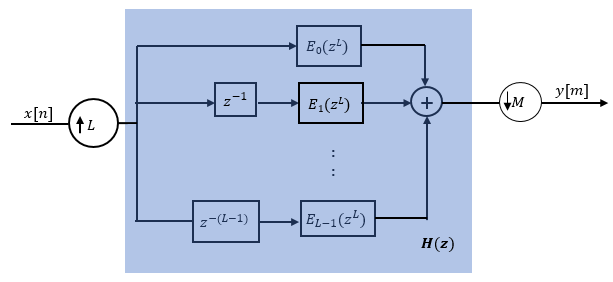Here is the multirate noble identity for interpolation.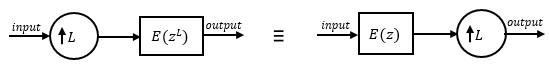Applying the noble identity for interpolation moves the upsampling operation to after the filtering operation. This move enables you to filter the signal at a lower rate.You can replace the upsampling operator, delay block, and the adder with a commutator switch. To account for the downsampler that follows, the switch moves in steps of size M. The switch receives the first sample from branch 0 and moves in the counter clockwise direction, each time skipping M−1 branches.

As an example, consider a rate converter with L set to 5 and M set to 3. The polyphase components are E0(z), E1(z), E2(z), E3(z), and E4(z). The switch starts on the first branch 0, skips branches 1 and 2, receives the next sample from branch 3, then skips branches 4 and 0, receives the next sample from branch 2, and so on. The sequence of branches from which the switch receives the data sample is [0, 3, 1, 4, 2, 0, 3, 1, ….].

The rate converter implements the L/M conversion by first applying the interpolation factor L to the incoming data, and using the commutator switch at the end to receive only 1 in M samples, effectively accounting for the dowsampling factor M. Hence, the sample rate at the output of the FIR rate converter is Lfs/M.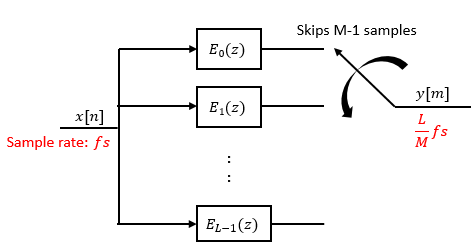Orfanidis, Sophocles J. Introduction to Signal Processing. Upper Saddle River, NJ: Prentice-Hall, 1996.

## SupportGet trial now